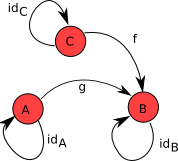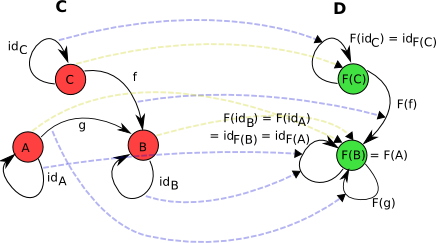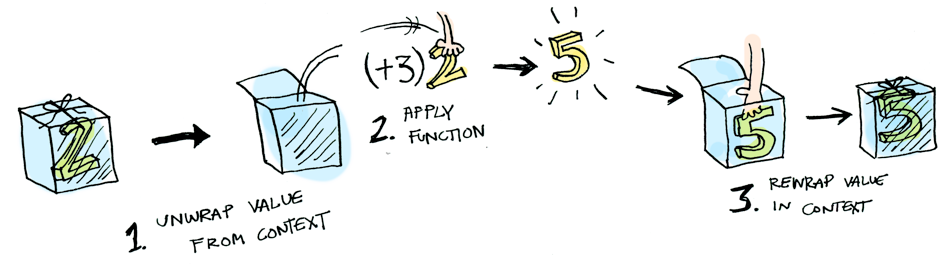A monad is just a monoid in the category of endofunctors, what’s the problem?

## Monoid

• 给定的类型 `T`
• 一个二元操作，`Op: (T,T) => T` 使得任意的 x, y, z ∈ T，满足 op(op(x,y), z) == op(x, op(y,z))
• 元单位 `Zero: T` 使得任意的 x ∈ T，满足 op(x, zero) == x and op(zero, x) == x

1. 若T为整数，则有 `(a+b)+c == a+(b+c)``0+n == n+0 == n`; `(a*b)*c == a*(b*c)``1*n == n*1 == n`
2. 若T为字符串，则有 `a+(b+c)==(a+b)+c; ""+s==s``s+"" == s`
3. 若T为数组，则有 `List(1,2)+List(3,4) == List(1,2,3,4)`
4. 若T为集合，则有 `Set(1,2,3)+Set(2,4) == Set(1,2,3,4)`

Monoid带来两个重要法则：

## 范畴

（1）一组对象
（2）一组态射（morphisms）。每个态射会绑定两个对象，假如f是从源对象A到目标对象B的态射，记作：`f：A -> B`
（3）态射组合。假如h是态射f和g的组合，记作：`h = g o f`#### 范畴公理

（1）态射的组合操作要满足结合律。记作：`f o (g o h) = (f o g) o h`
（2）对任何一个范畴 C，其中任何一个对象A一定存在一个单位态射，`id_A: A => A`。并且对于态射`g：A => B``id_B o g = g = g o id_A`
（3）态射在组合操作下是闭合的。所以如果存在态射`f: A => B``g: B => C`，那么范畴中必定存在态射 `h: A => C` 使得 `h = g o f``f``g` 都是态射，所以我们一定能够对它们进行组合并得到范畴中的另一个态射。那么哪一个是态射 `f o g` 呢？唯一的选择就是 `id_A` 了。类似地，`g o f=id_B`

## 函子(Functor)

#### 函子定义#### 函子公理

（1）给定一个对象 A 上的单位态射Id_A ， F(Id_A) 必须也是 F(A) 上的单位态射，也就是说：`F(Id_A) = Id_(F(A))`
（2）函子在态射组合上必须满足分配律，也就是说：`F(f o g) = F(f) o F(g)`

1. 函数表达的映射关系在类型上体现在**特定类型(proper type)**上。
1. 函子表达的映射关系，则体现在**高阶函数(high-order function)**上(确切来说是范畴)。

1. 将C1中的类型 T 映射为 C2 中的 `List[T] : T => List[T]`
2. 将C1中的函数 f 映射为 C2 中的 函数`fm : (A => B) => (List[A] => List[B])`

## 自函子(Endofunctor)

1. `unit: x -> M[x]`
2. `join/bind: M[M[x]] -> M[x]`Monad在类库中总是和`implicity`一起使用，我们不做这么复杂，假设自函子范畴是`List`，实现：